# Compare Speaker Separation Models

Compare the performance, size, and speed of deep learning speaker separation models.

### Introduction

Speaker separation is a challenging and critical speech processing task. Modern speaker separation methods use deep learning to achieve strong results. In this example, you compare four speaker separation models:

• Conv-TasNet model trained to discriminate two speakers 

• Conv-TasNet model trained to discriminate one speaker and put the "rest" in a separate channel. This type of model is known as "one-and-rest".  

• SepFormer model trained to discriminate two speakers 

You can find the training recipes for the time-frequency masking model and the 2-speaker ConvTas-Net model in Cocktail Party Source Separation Using Deep Learning Networks and End-to-End Deep Speaker Separation, respectively. You can perform speaker separation using the one-and-rest Conv-TasNet (Conv-TasNet OR) model and the SepFormer model with the `separateSpeakers` function.

To spot-check model performance, load test data consisting of two speakers and their mix. Listen to the speakers individually and mixed. Plot the mix and individual speakers using the supporting function, `plotSpeakerSeparation`.

```[audioIn,fs] = audioread("MultipleSpeakers-16-8-4channel-5secs.flac"); t1 = audioIn(:,2); t2 = audioIn(:,3); x = t1 + t2; x = x/max(abs(x)); plotSpeakerSeparation(t1,t2,x)```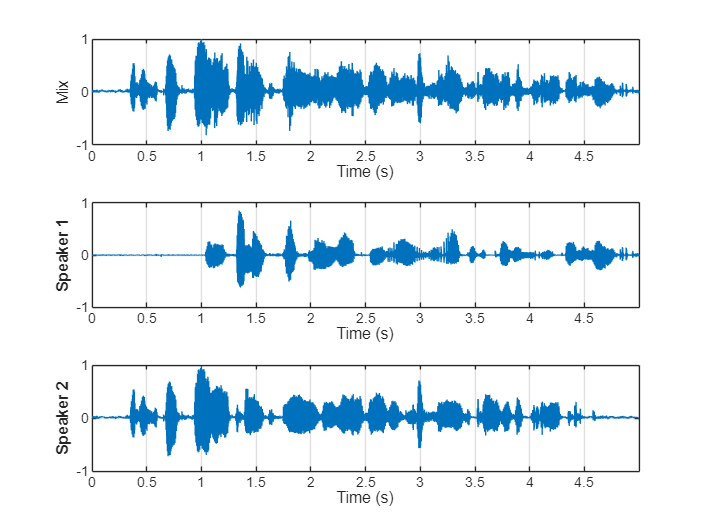```sound(t1,fs),pause(5) sound(t2,fs),pause(5) sound(x,fs),pause(5)```

Load the pretrained speaker separation weights for the time-frequency masking model. The inference model is defined in the supporting function `separateSpeakersTimeFrequency`. To examine and train this model, see Cocktail Party Source Separation Using Deep Learning Networks.

```downloadFolder = matlab.internal.examples.downloadSupportFile("audio","CocktailPartySourceSeparation.zip"); dataFolder = tempdir; unzip(downloadFolder,dataFolder) tfNetFolder = fullfile(dataFolder,"CocktailPartySourceSeparation");```

Separate the mixed test signal and then plot and listen to the results.

```y = separateSpeakersTimeFrequency(x,tfNetFolder); plotSpeakerSeparation(t1,t2,x,y(:,1),y(:,2))```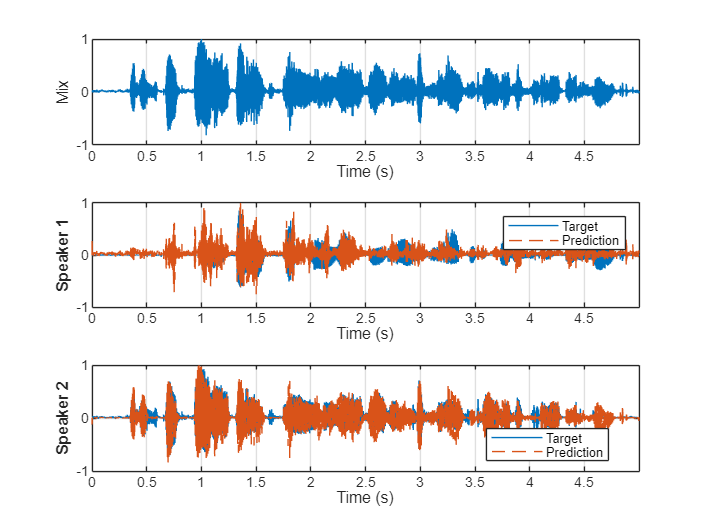```sound(y(:,1),fs),pause(5) sound(y(:,2),fs),pause(5)```

#### Conv-TasNet

Load the pretrained speaker separation weights for the Conv-TasNet model. The inference model is defined in the supporting function `separateSpeakersConvTasNet`. To examine and train this model, see End-to-End Deep Speaker Separation.

```downloadFolder = matlab.internal.examples.downloadSupportFile("audio","speechSeparation.zip"); dataFolder = tempdir; unzip(downloadFolder,dataFolder) convtasNetFolder = fullfile(dataFolder,"speechSeparation");```

Separate the mixed test signal and then plot and listen to the results.

```y = separateSpeakersConvTasNet(x,convtasNetFolder); plotSpeakerSeparation(t1,t2,x,y(:,1),y(:,2));```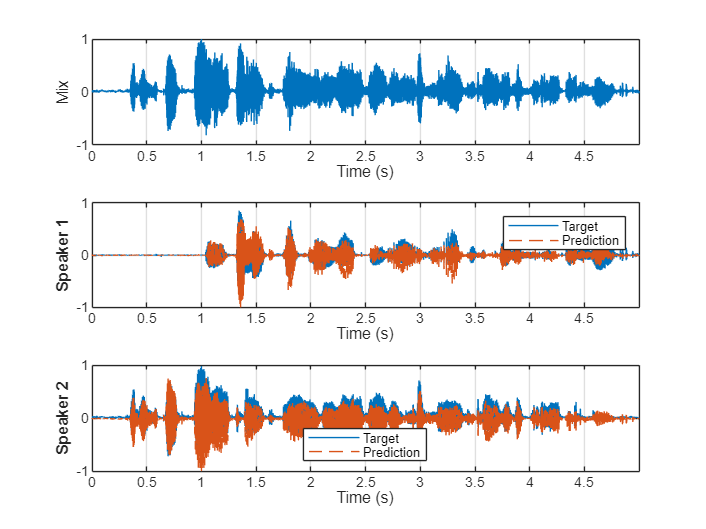```sound(y(:,1),fs),pause(5) sound(y(:,2),fs),pause(5)```

#### Conv-TasNet with One-and-Rest Permutation Invariant Training

The `separateSpeakers` function uses three models under-the-hood: a 2-speaker SepFormer model, a 3-speaker SepFormer model, and a one-and-rest Conv-TasNet model. To use the one-and-rest Conv-TasNet model, specify `NumSpeakers` as `1` or do not specify the `NumSpeakers`. When `NumSpeakers` is not specified, the function passes the "rest" from the separation back through the model until no more speakers are detected. For the purposes of this example, call `separateSpeakers` twice with `NumSpeakers=1` for both calls.

Separate the mixed test signal and then plot and listen to the results. If you have not downloaded the required files to use `separateSpeakers`, an error is thrown with the link to the download.

```[y1,r] = separateSpeakers(x,fs,NumSpeakers=1); [y2,r] = separateSpeakers(r,fs,NumSpeakers=1); plotSpeakerSeparation(t1,t2,x,y1,y2)```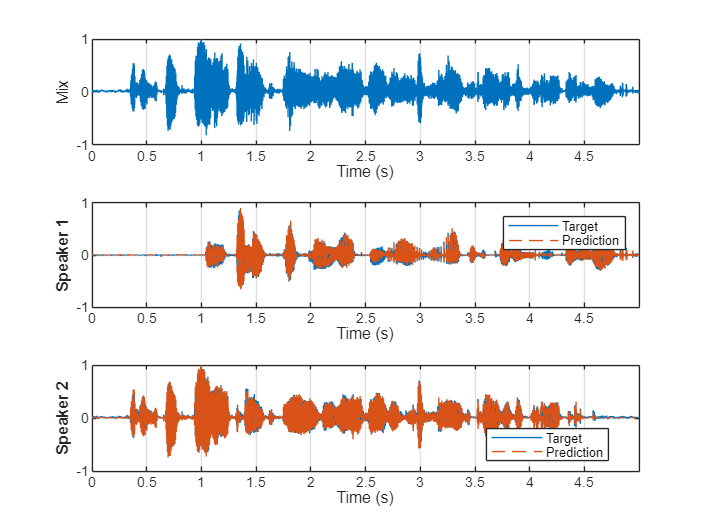```sound(y1,fs),pause(5) sound(y2,fs),pause(5)```

#### SepFormer

Call `separateSpeakers` with `NumSpeakers=2` to perform speaker separation using the 2-speaker SepFormer model.

Separate the mixed test signal and then plot and listen to the results.

```y = separateSpeakers(x,fs,NumSpeakers=2); plotSpeakerSeparation(t1,t2,x,y(:,1),y(:,2))```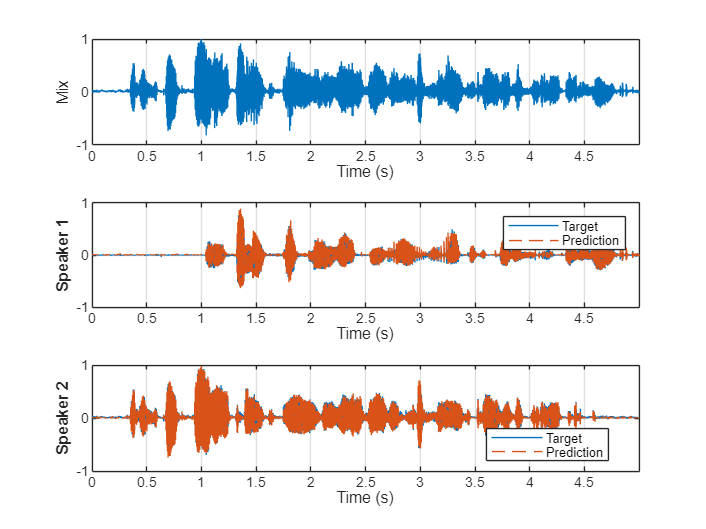```sound(y(:,1),fs),pause(5) sound(y(:,2),fs),pause(5)```

### Compare Models

Compare the computation time, model size, and performance of the models.

#### Computation Time

To compare execution times for different duration inputs, use the supporting function `compareComputationTime`. If the execution time is less than the input duration, then the model can run in real time (without dropping samples).

```compareComputationTime(DurationToTest=[1,5,10], ... CompareCPU=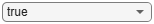true, ... CompareGPU=true, ... TimeFrequencyMaskNetFolder=tfNetFolder, ... ConvTasNetFolder=convtasNetFolder)```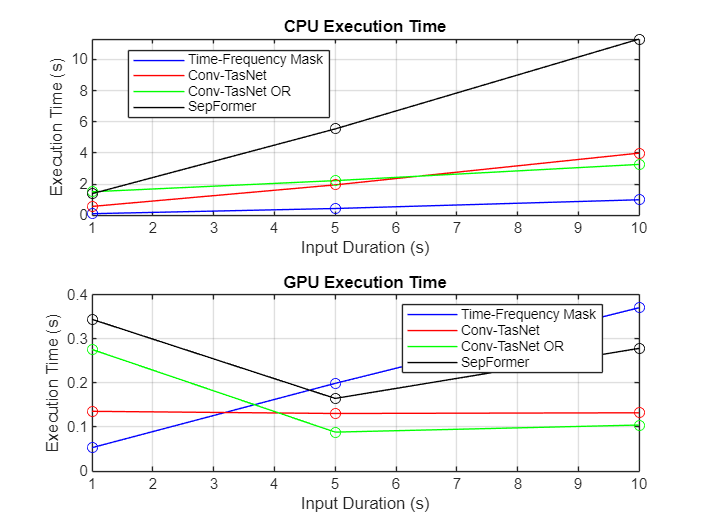#### Model Size

Compare the size of all models. Note that the Conv-TasNet model trained in the example and the Conv-TasNet OR model provided with the `separateSpeakers` function are quite different in size. In addition to different loss functions and training recipes, Conv-TasNet OR and Conv-TasNet are both variations on the architecture described in . Most noticeably, the Conv-TasNet OR model uses 24 convolutional blocks while the example Conv-TasNet model uses 32.

```timefrequency_size = dir(fullfile(tfNetFolder,"CocktailPartyNet.mat")).bytes/1e6; convtasnet_size = dir(fullfile(convtasNetFolder,"paramsBest.mat")).bytes/1e6; convtasnet_or_size = dir(which("convtasnet-librimix-orpit.mat")).bytes/1e6; sepformer_size = dir(which("sepformer-libri2mix-upit.mat")).bytes/1e6; n = categorical(["Time-Frequency Mask","Conv-TasNet","Conv-TasNet OR","SepFormer"]); n = reordercats(n,["Time-Frequency Mask","Conv-TasNet","Conv-TasNet OR","SepFormer"]); figure bar(n,[timefrequency_size,convtasnet_size,convtasnet_or_size,sepformer_size]) grid on ylabel("Size (MB)") title("Disk Memory")```#### Compare Scale Invariant Signal to Noise Ratio (SNR)

To compare model performance, download the LibriSpeech  test-clean dataset. The dataset consists of files of single speakers reading.

```downloadDatasetFolder = tempdir; datasetFolder = fullfile(downloadDatasetFolder,"LibriSpeech","test-clean"); filename = "test-clean.tar.gz"; url = "http://www.openSLR.org/resources/12/" + filename; if ~datasetExists(datasetFolder) gunzip(url,downloadDatasetFolder); unzippedFile = fullfile(downloadDatasetFolder,filename); untar(unzippedFile{1}(1:end-3),downloadDatasetFolder); end ads = audioDatastore(datasetFolder,IncludeSubfolders=true);```

Test the model scale-invariant signal-to-noise ratio (SI-SNR)  performances on a sampling of the dataset. SI-SNR is a popular objective metric for the quality of speaker separation algorithms. If a GPU and Parallel Computing Toolbox™ are available, use the GPU to speed up processing.

The `testModel` supporting function combines randomly selected audio files, mixes them, passes the mixed data through the specified model, and then calculates the permutation-invariant SI-SNR.

The SepFormer model achieves the best results (higher SNR is better).

```tf_sisnr = testModel(ads,@(x)separateSpeakersTimeFrequency(x,tfNetFolder),UseGPU=canUseGPU); convtasnet_sisnr = testModel(ads,@(x)separateSpeakersConvTasNet(x,convtasNetFolder),UseGPU=canUseGPU); convtasnet_orpit_sisnr = testModel(ads,@(x)separateSpeakers(x,8e3,NumSpeakers=1),UseGPU=canUseGPU,OneAndRest=true); sepformer_sisnr = testModel(ads,@(x)separateSpeakers(x,8e3,NumSpeakers=2),UseGPU=canUseGPU); figure bar(n,[tf_sisnr,convtasnet_sisnr,convtasnet_orpit_sisnr,sepformer_sisnr]) grid on ylabel("SI-SNR") title("Separation Performance (Test Dataset)")```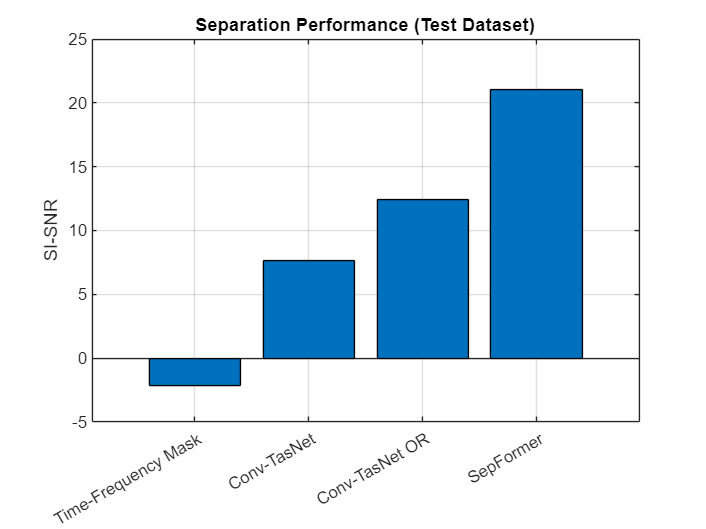### Supporting Functions

#### Test Model

```function testSISNR = testModel(ads,model,options) %testModel Calculate SISNR over dataset arguments ads model options.OneAndRest = false options.UseGPU = false options.NumTestPoints = 50 options.TestDuration = [] options.SignalRatio = [0.6 0.75 0.85 1] end total_sisnr = zeros(options.NumTestPoints,1); fn = ads.Files; spkids = filenames2labels(fn,ExtractBefore="-"); rng default for ii = 1:options.NumTestPoints % Choose a random file for speaker 1 idx1 = randi(numel(fn)); fn1 = fn{idx1}; % Choose another random file for speaker 2, exclude speaker 1 from % possibilities spk1 = spkids(idx1); fnsubset = fn(spkids~=spk1); idx2 = randi(numel(fnsubset)); fn2 = fnsubset{idx2}; % Read audio file and resample to 8 kHz. [t1,fs1] = audioread(fn1); [t2,fs2] = audioread(fn2); t1 = resample(t1,8e3,fs1); t2 = resample(t2,8e3,fs2); % Make files the same length if isempty(options.TestDuration) N = min(numel(t1),numel(t2)); else N = 8e3*options.TestDuration; end t1 = trimOrPad(t1,N); t2 = trimOrPad(t2,N); % Scale audio to abs max of 1 t1 = t1./max(abs(t1)); t2 = t2./max(abs(t2)); % Scale the second speaker according to the scaling ratio t2 = options.SignalRatio(randi(numel(options.SignalRatio)))*t2; % Mix x = t1 + t2; x = x./max(abs(x)); % Use GPU if requested if options.UseGPU x = gpuArray(x); end % Pass signal through model if options.OneAndRest [y1,r] = model(x); y2 = model(r); else y = model(x); y2 = y(:,2); y1 = y(:,1); end [y1,y2] = gather(y1,y2); % Perform permutation invariant signal to noise ratio sisnr = uPIT(t1,y1,t2,y2); total_sisnr(ii) = sisnr; end testSISNR = mean(total_sisnr); end```

#### Plot Speaker Separation

```function plotSpeakerSeparation(t1,t2,x,y1,y2) %plotSpeakerSeparation Plot the ground truth and predictions arguments t1 t2 x y1 = [] y2 = [] end fs = 8e3; timeVector = ((0:size(t1,1)-1)/fs)'; tiledlayout(3,1) nexttile() plot(timeVector,x) xlabel("Time (s)") ylabel("Mix") grid on xlim tight ylim([-1 1]) % Match the targets and predictions based on which set of pairs results in % the best SI-SNR if ~isempty(y1) [~,reverseOrder] = uPIT(t1,y1,t2,y2); if reverseOrder ytemp = y1; y1 = y2; y2 = ytemp; end end nexttile() if ~isempty(y1) plot(timeVector,t1,"-",timeVector,y1,"--") legend("Target","Prediction") else plot(timeVector,t1) end ylabel("Speaker 1",FontWeight="bold") xlabel("Time (s)") grid on xlim tight ylim([-1 1]) nexttile() if ~isempty(y2) plot(timeVector,t2,"-",timeVector,y2,"--") legend("Target","Prediction",Location="best") else plot(timeVector,t2) end ylabel("Speaker 2",FontWeight="bold") xlabel("Time (s)") grid on xlim tight ylim([-1 1]) end```

#### Scale Invariant Signal to Noise Ratio (SISNR)

```function z = SISNR(t,y) %SISNR Compute SI-SNR t = t - mean(t); y = y - mean(y); t = sum(t.*y) .* y ./ (sum(y.^2)+eps); z = 20*log((sqrt(sum(t.^2))+eps)./sqrt((sum((y-t).^2))+eps))/log(10); end```

#### Utterance-Level Permutation Invariant Training (uPIT)

```function [m,reverseOrder] = uPIT(t1,y1,t2,y2) %uPIT Compute utterance-level permutation invariant training v1 = SISNR(t1,y1); v2 = SISNR(t2,y2); m1 = mean([v1;v2]); v1 = SISNR(t1,y2); v2 = SISNR(t2,y1); m2 = mean([v1;v2]); [m,idx] = max([m1,m2]); reverseOrder = idx==2; end```

#### Separate Speakers Using Time-Frequency Masking

```function output = separateSpeakersTimeFrequency(mix,pathToNet) %separateSpeakersTimeFrequency STFT-based speaker separation function persistent CocktailPartyNet if isempty(CocktailPartyNet) s = load(fullfile(pathToNet,"CocktailPartyNet.mat")); CocktailPartyNet = s.CocktailPartyNet; end WindowLength = 128; FFTLength = 128; OverlapLength = 128-1; win = hann(WindowLength,"periodic"); % Downsample to 4 kHz mixR = resample(mix,1,2); P0 = stft(mixR, ... Window=win, ... OverlapLength=OverlapLength,... FFTLength=FFTLength, ... FrequencyRange="onesided"); P = log(abs(P0) + eps); MP = mean(P(:)); SP = std(P(:)); P = (P-MP)/SP; seqLen = 20; PSeq = zeros(1 + FFTLength/2,seqLen,1,0); seqOverlap = seqLen; loc = 1; while loc < size(P,2)-seqLen PSeq(:,:,:,end+1) = P(:,loc:loc+seqLen-1); %#ok loc = loc + seqOverlap; end PSeq = reshape(PSeq, [1 1 (1 + FFTLength/2)*seqLen size(PSeq,4)]); estimatedMasks = predict(CocktailPartyNet,PSeq); estimatedMasks = estimatedMasks.'; estimatedMasks = reshape(estimatedMasks,1 + FFTLength/2,numel(estimatedMasks)/(1 + FFTLength/2)); mask1 = estimatedMasks; mask2 = 1 - mask1; P0 = P0(:,1:size(mask1,2)); P_speaker1 = P0.*mask1; speaker1 = istft(P_speaker1, ... Window=win, ... OverlapLength=OverlapLength,... FFTLength=FFTLength, ... ConjugateSymmetric=true,... FrequencyRange="onesided"); speaker1 = speaker1 / max(abs(speaker1)); P_speaker2 = P0.*mask2; speaker2 = istft(P_speaker2, ... Window=win, ... OverlapLength=OverlapLength,... FFTLength=FFTLength, ... ConjugateSymmetric=true,... FrequencyRange="onesided"); speaker2 = speaker2/max(speaker2); speaker1 = resample(double(speaker1),2,1); speaker2 = resample(double(speaker2),2,1); N = numel(mix) - numel(speaker1); mixToAdd = mix(end-N+1:end); speaker1 = [speaker1;mixToAdd]; speaker2 = [speaker2;mixToAdd]; output = [speaker1,speaker2]; end ```

#### Separate Speakers Using uPIT ConvTasNet

```function output = separateSpeakersConvTasNet(input,netFolder) %separateSpeakersConvTasNet Separate two speaker signals from a mixture % input using 2-speaker Conv-TasNet. persistent learnables states if isempty(learnables) M = load(fullfile(netFolder,"paramsBest.mat")); learnables = M.learnables; states = M.states; end if ~isdlarray(input) input = dlarray(input,"SCB"); end x = dlconv(input,learnables.Conv1W,learnables.Conv1B,Stride=10); x = relu(x); x0 = x; x = x - mean(x,2); x = x./sqrt(mean(x.^2, 2) + 1e-5); x = x.*learnables.ln_weight + learnables.ln_bias; encoderOut = dlconv(x,learnables.Conv2W,learnables.Conv2B); for index = 1:32 encoderOut = convBlock(encoderOut,index-1,learnables.Blocks(index),states(index)); end masks = dlconv(encoderOut,learnables.Conv3W,learnables.Conv3B); masks = relu(masks); mask1 = masks(:,1:256,:); mask2 = masks(:,257:512,:); out1 = x0.*mask1; out2 = x0.*mask2; weights = learnables.TransConv1W; bias = learnables.TransConv1B; output2 = dltranspconv(out1,weights,bias,Stride=10); output1 = dltranspconv(out2,weights,bias,Stride=10); output1 = gather(extractdata(output1)); output2 = gather(extractdata(output2)); output1 = output1./max(abs(output1)); output2 = output2./max(abs(output2)); output1 = trimOrPad(output1,numel(input)); output2 = trimOrPad(output2,numel(input)); output = [output1,output2]; end```

#### ConvTasNet - Conv Block

```function output = convBlock(input,count,learnables,state) %convBlock - Convolutional block for ConvTasNet % Conv: conv1Out = dlconv(input,learnables.Conv1W,learnables.Conv1B); % PRelu: conv1Out = relu(conv1Out) - learnables.Prelu1.*relu(-conv1Out); % BatchNormalization: batchOut = batchnorm(conv1Out,learnables.BN1Offset,learnables.BN1Scale,state.BN1Mean,state.BN1Var); % Conv: padding = [1 1] * 2^(mod(count,8)); dilationFactor = 2^(mod(count,8)); convOut = dlconv(batchOut,learnables.Conv2W,learnables.Conv2B,DilationFactor=dilationFactor,Padding=padding); % PRelu: convOut = relu(convOut) - learnables.Prelu2.*relu(-convOut); % BatchNormalization: batchOut = batchnorm(convOut,learnables.BN2Offset,learnables.BN2Scale,state.BN2Mean,state.BN2Var); % Conv: output = dlconv(batchOut,learnables.Conv3W,learnables.Conv3B); % Skip connection output = output + input; end ```

#### Trim or Pad Audio Signal to Desired Length

```function y = trimOrPad(x,n) %trimOrPad Trim or pad to desired length % Trim or expand to match desired size if size(x,1)>=n % Choose a random starting index such that you still have numSamples % after indexing the noise. start = randi(size(x,1) - n + 1); y = x(start:start+n-1); else numReps = ceil(n/size(x,1)); temp = repmat(x,numReps,1); start = randi(size(temp,1) - n + 1); y = temp(start:start+n-1); end end```

#### Compare Execution Time

```function compareComputationTime(options) %compareComputationTime Compare computation time arguments options.DurationToTest options.CompareCPU options.CompareGPU options.TimeFrequencyMaskNetFolder options.ConvTasNetFolder end fs = 8e3; dur = options.DurationToTest; if options.CompareCPU tf.CPU = zeros(numel(dur),1); convtas.CPU = zeros(numel(dur),1); convtas_orpit.CPU = zeros(numel(dur),1); sepformer.CPU = zeros(numel(dur),1); for ii = 1:numel(dur) x = pinknoise(dur(ii)*fs,"single"); tf.CPU(ii) = timeit(@()separateSpeakersTimeFrequency(x,options.TimeFrequencyMaskNetFolder)); convtas.CPU(ii) = timeit(@()separateSpeakersConvTasNet(x,options.ConvTasNetFolder)); convtas_orpit.CPU(ii) = timeit(@()separateSpeakers(x,8e3,NumSpeakers=1,ConserveEnergy=false)); sepformer.CPU(ii) = timeit(@()separateSpeakers(x,8e3,NumSpeakers=2,ConserveEnergy=false)); end convtas_orpit.CPU = 2*convtas_orpit.CPU; % Double to adjust for two-passes of one-and-rest. end if options.CompareGPU tf.GPU = zeros(numel(dur),1); convtas.GPU = zeros(numel(dur),1); convtas_orpit.GPU = zeros(numel(dur),1); sepformer.GPU = zeros(numel(dur),1); for ii = 1:numel(dur) x = gpuArray(pinknoise(dur(ii)*fs,"single")); tf.GPU(ii) = gputimeit(@()separateSpeakersTimeFrequency(x,options.TimeFrequencyMaskNetFolder)); convtas.GPU(ii) = gputimeit(@()separateSpeakersConvTasNet(x,options.ConvTasNetFolder)); convtas_orpit.GPU(ii) = gputimeit(@()separateSpeakers(x,8e3,NumSpeakers=1,ConserveEnergy=false)); sepformer.GPU(ii) = gputimeit(@()separateSpeakers(x,8e3,NumSpeakers=2,ConserveEnergy=false)); end convtas_orpit.GPU = 2*convtas_orpit.GPU; % Double to adjust for two-passes of one-and-rest. end numTiles = double(options.CompareCPU)+double(options.CompareGPU); tlh = tiledlayout(numTiles,1); environments = ["CPU","GPU"]; environments = environments([options.CompareCPU,options.CompareGPU]); for ii = 1:numel(environments) nexttile(tlh) ee = environments(ii); plot(dur,tf.(ee),'b',dur,convtas.(ee),'r',dur,convtas_orpit.(ee),'g',dur,sepformer.(ee),'k', ... dur,tf.(ee),'bo',dur,convtas.(ee),'ro',dur,convtas_orpit.(ee),'go',dur,sepformer.(ee),'ko') legend("Time-Frequency Mask","Conv-TasNet","Conv-TasNet OR","SepFormer",Location="best") xlabel("Input Duration (s)") ylabel("Execution Time (s)") title(ee + " Execution Time") grid on end end```

### References

 Luo, Yi, and Nima Mesgarani. "Conv-TasNet: Surpassing Ideal Time-Frequency Magnitude Masking for Speech Separation." IEEE/ACM Transactions on Audio, Speech, and Language Processing 27, no. 8 (August 2019): 1256-66. https://doi.org/10.1109/TASLP.2019.2915167.

 Simpson, Andrew J. R. "Probabilistic Binary-Mask Cocktail-Party Source Separation in a Convolutional Deep Neural Network." arXiv.org, March 24, 2015. https://arxiv.org/abs/1503.06962.

 Panayotov, Vassil, Guoguo Chen, Daniel Povey, and Sanjeev Khudanpur. "Librispeech: An ASR Corpus Based on Public Domain Audio Books." In 2015 IEEE International Conference on Acoustics, Speech and Signal Processing (ICASSP), 5206-10. South Brisbane, Queensland, Australia: IEEE, 2015. https://doi.org/10.1109/ICASSP.2015.7178964.

 Subakan, Cem, Mirco Ravanelli, Samuele Cornell, Mirko Bronzi, and Jianyuan Zhong. "Attention Is All You Need In Speech Separation." In ICASSP 2021 - 2021 IEEE International Conference on Acoustics, Speech and Signal Processing (ICASSP), 21-25. Toronto, ON, Canada: IEEE, 2021. https://doi.org/10.1109/ICASSP39728.2021.9413901.

 Takahashi, Naoya, Sudarsanam Parthasaarathy, Nabarun Goswami, and Yuki Mitsufuji. "Recursive Speech Separation for Unknown Number of Speakers." In Interspeech 2019, 1348-52. ISCA, 2019. https://doi.org/10.21437/Interspeech.2019-1550.

 Roux, Jonathan Le, et al. "SDR – Half-Baked or Well Done?" ICASSP 2019 - 2019 IEEE International Conference on Acoustics, Speech and Signal Processing (ICASSP), IEEE, 2019, pp. 626–30. DOI.org (Crossref), https://doi.org/10.1109/ICASSP.2019.8683855.